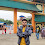# HackerRank Boxes through a Tunnel solution in c programming

In this HackerRank Boxes through a Tunnel c programming problem solution You are transporting some boxes through a tunnel, where each box is a parallelepiped, and is characterized by its length, width and height.

The height of the tunnel 41 feet and the width can be assumed to be infinite. A box can be carried through the tunnel only if its height is strictly less than the tunnel's height. Find the volume of each box that can be successfully transported to the other end of the tunnel. Note: Boxes cannot be rotated.

## HackerRank Boxes through a tunnel problem solution in c programming.

```#include <stdio.h>
struct Box
{
int length, width, height;
};

int volume(struct Box box)
{
return box.length*box.width*box.height;
}

int lower(struct Box box, int maxHeight)
{
return box.height < maxHeight;
}

int main()
{
int n;
scanf("%d", &n);
struct Box boxes;
for (int i = 0; i < n; i++)
scanf("%d%d%d", &boxes[i].length, &boxes[i].width, &boxes[i].height);
for (int i = 0; i < n; i++)
if (lower(boxes[i], 41))
printf("%d\n", volume(boxes[i]));
return 0;
}```

## Second solution

```#include <stdio.h>
#include <stdlib.h>
#define MAX_HEIGHT 41

typedef struct {
int height;
int length;
int width;
}box;

int get_volume(box b) {
return b.length * b.height * b.width;
}

int is_lower_than_max_height(box b) {
return b.height < 41 ? 1 : 0;
}

int main()
{
int n;
scanf("%d", &n);
box *boxes = malloc(n * sizeof(box));
for (int i = 0; i < n; i++) {
scanf("%d%d%d", &boxes[i].length, &boxes[i].width, &boxes[i].height);
}
for (int i = 0; i < n; i++) {
if (is_lower_than_max_height(boxes[i])) {
printf("%d\n", get_volume(boxes[i]));
}
}
return 0;
}```

1.1.